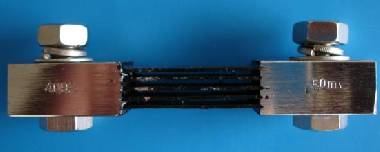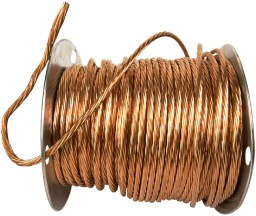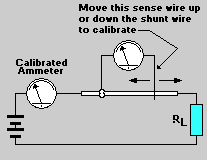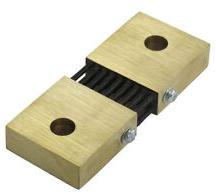# Make a Shunt Resistor

A shunt resistor (pictured below) is a high-precision resistor of very low resistance used by anammeter to measure the current flowing through an electric circuit. Shunts are available in a range of sizes from under one Amp to many hundreds and thousands of Amps, but they can be very expensive.Fortunately it is possible to make your own shunt resistor from nothing more than a length of copper wire. While it will only be accurate to within 10%, and its accuracy will fall with changes in temperature much more so than would be the case with a real shunt resistor – making your own shunts is very cheap and easy and can also be an interesting education project.

### Example DIY Shunt Resistor

In this example we will look at making a 0-10 Amp shunt resistor with a voltage drop of 10mV at 10 Amps. This means that if 10 Amps of current are flowing through the shunt resistor, the voltage measured between the two ends of it will be 10mV (0.010 Volts). Therefore using a standard multimeter or 0-10 mV voltmeter it will be easy to read the numerical value of the current (and ignore the units displayed) – i.e. if the voltmeter reads 6 mV we know this corresponds to 6 Amps of current, 2.4 mV corresponds to 2.4 Amps and so on since the voltage across the shunt resistor is directly proportional to the current flowing through it.

So, what do we need the resistance of our shunt resistor to be for a 10mv voltage drop at 10 Amps? Using Ohm’s Law, the voltage drop (0.01V) divided by the current (10A) gives the restance of the shuntwhich equals 0.001 Ohms. Therefore our shunt resistor needs simply to be 0.001 Ohm resistor.

### Resistance of Copper Wire

Copper wire has a resistance which depends upon its length and its diameter. The thicker the wire, the lower its resistance; the longer the wire, the greater its resistance. For example 24 AWG copper wire (see our wire size converter for more information about AWG) has a known resistance of 25.67 Ohms per 1000 feet of length at 25 degrees celcius.

Click here to view a comprehensive table of bare copper wire resistance values for different diameters of wire.### Using Copper Wire to make a 0.001 Ohm Shunt Resistor

10 AWG copper wire (2.59mm diameter) for example has a resistance of 0.9989 Ohms per 1,000 feet. Therefore our 0.001 Ohm resisitor would require 1000 * 0.001/0.9989 = 1.001 feet of 10 AWG copper wire.
12 AWG copper wire (2.05mm diameter) has a resistance of 1.5883 Ohms per 1,000 feet and so 1000 * 0.001/1.5883 = 0.63 feet of 12 AWG wire would be required for the 0.001 Ohm resistor.

Wire Length (feet) =
 1000 * Target Resistance (Ohms) Ohms per 1,000 feet

### Basic Shunt Resistor

In the simplest case copper wire of the calculated length is simply soldered in series into the circuit to be measured and the positive and negative leads of the voltmeter connected to those joints. Unfortunately this would not be a very accurate ammeter because the resistance of the soldered joints can affect the results.

### Calibrated Shunt Resistor

The shunt resistor will be much more accurate if it is calibrated by comparing the results it gives to those given by a precise ammeter. An ammeter should be connected into the circuit so the true value of the current flowing through it can be measured and this reading compared to that given by the voltmeter and copper wire shunt combination.A length of copper wire a good 25% longer than calculated above should be joined in series with the circuit to be measured as shown in the above schematic. One of the leads of the voltmeter should then be soldered near to one end of the copper wire. The remaining voltmeter lead can then be moved along the length of copper wire until the voltmeter reading is the same as that of the ammeter. The voltmeter lead can then be soldered into place leaving a calibrated relatively accurate homemade shunt resistor.

Thick wire has lower resistance than thin wire therefore a more accurate shunt can be made with thick wire, but it will be more expensive. However, remember that to measure large currents, thick wire mustbe used as thin wire will overheat.

### 2014 Article Update – Buying Shunt Resistors

One of the major things which has changed since the article was first published in 2007 is the increasing availability of well priced shunt resistors.Pictured above for example is a 1000 Amp 75 mV shunt resistor priced at just £12 delivered to the UK from Hong Kong. Click here for a wide selection of shunt resistors with prices starting at just £2 each delivered.